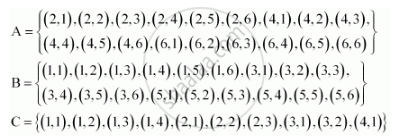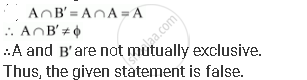# Two Dice Are Thrown. the Events A, B and C Are as Follows: a and B' Are Mutually Exclusive - Mathematics

Two dice are thrown. The events A, B and C are as follows:

A: getting an even number on the first die.

B: getting an odd number on the first die.

C: getting the sum of the numbers on the dice ≤ 5

A and B' are mutually exclusive

#### SolutionIs there an error in this question or solution?
Chapter 16: Probability - Exercise 16.2 [Page 393]

#### APPEARS IN

NCERT Class 11 Mathematics
Chapter 16 Probability
Exercise 16.2 | Q 7.5 | Page 393

Share